# HSSlive: Plus One & Plus Two Notes & Solutions for Kerala State Board

## AP Board Class 7 Maths Chapter 7 Ratio and Proportion Ex 7.5 Textbook Solutions PDF: Download Andhra Pradesh Board STD 7th Maths Chapter 7 Ratio and Proportion Ex 7.5 Book AnswersAP Board Class 7 Maths Chapter 7 Ratio and Proportion Ex 7.5 Textbook Solutions PDF: Download Andhra Pradesh Board STD 7th Maths Chapter 7 Ratio and Proportion Ex 7.5 Book Answers

## Andhra Pradesh State Board Class 7th Maths Chapter 7 Ratio and Proportion Ex 7.5 Books Solutions

 Board AP Board Materials Textbook Solutions/Guide Format DOC/PDF Class 7th Subject Maths Chapters Maths Chapter 7 Ratio and Proportion Ex 7.5 Provider Hsslive

2. Click on the Andhra Pradesh Board Class 7th Maths Chapter 7 Ratio and Proportion Ex 7.5 Answers.
3. Look for your Andhra Pradesh Board STD 7th Maths Chapter 7 Ratio and Proportion Ex 7.5 Textbooks PDF.
4. Now download or read the Andhra Pradesh Board Class 7th Maths Chapter 7 Ratio and Proportion Ex 7.5 Textbook Solutions for PDF Free.

## AP Board Class 7th Maths Chapter 7 Ratio and Proportion Ex 7.5 Textbooks Solutions with Answer PDF Download

Find below the list of all AP Board Class 7th Maths Chapter 7 Ratio and Proportion Ex 7.5 Textbook Solutions for PDF’s for you to download and prepare for the upcoming exams:

Question 1.
Rekha bought a wrist watch for ₹ 2250 and sold it for ₹ 1890. Then find her loss or gain percentage?Given cost price of wrist watch = ₹ 2250
Selling price of wrist watch = ₹ 1890
C.P > S.P, then Rekha got loss.
Loss = C.P – S.P = 2250 – 1890 = ₹ 360

We know loss percent =  Loss  C.P  × 100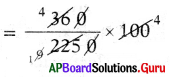∴ Loss percentage 16%.

Question 2.
A shopkeeper buys a toy for ₹ 250 and sells it for ₹ 300. Find his gain or loss percentage?Given cost price of a toy = ₹ 250
Selling price of a toy = ₹ 300
C.P < S.P, then Shopkeeper got profit.
Profit = S.P – C.P
= 300 – 250 = ₹ 50

We know profit percent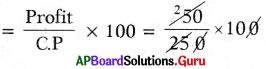∴ Profit percentage = 20%

Question 3.
The cost price of a chair ₹ 480. 1f he sold at a profit of 10%. what would be selling price of it’?
Method: 1
Given cost price of a chair = ₹ 480
Profit percent = 10%
Profit = 10% of ₹ 480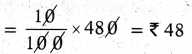Selling price = C.P + Profit
= ₹ 480 + ₹ 48
∴ Selling price = ₹ 528

Method: 2
C.P = 100% = ₹ 480
Selling it 10% gain.
= S.P = 100% + 10% =?
z 100% = ₹ 480
110% =?
S.P = 110100 × 480= ₹ 528
∴ Selling price = ₹ 528

Question 4.
If Sharma purchased a car for ₹ 350000. After two years he sold at a loss of 12%. Find its selling price’?
Given cost price of a car = ₹ 350000
Loss = 12 %
Loss 12% of ₹ 350000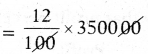Loss = ₹ 42000
Selling price = CP – Loss
= 350000 – 42000
∴ Selling price of a car = ₹ 3,08,000

Question 5.
A shopkeeper buys wooden tables each at ₹ 2800 and expends ₹ 400 on each table for painting. If he sells it at a cost of ₹ 4000. Find his profit percentage?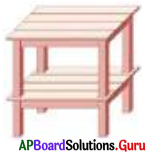Given cost price of wooden table = ₹ 2800
Amount spent on painting = ₹ 400
Effective cost price of wooden table
= ₹ 2800 + ₹ 400 = ₹ 3200

Selling price wooden table = 4000
C.P < SP, then shopkeeper got profit.
Profit = S.P – C.P
= ₹ 4000 – ₹ 3200 = ₹ 800

We know profit percentage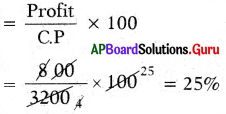∴ Profit percentage = 25%

Question 6.
In a garment shop a saree is sold at a cost of ₹ 1800 after a profit of ₹ 600. Find the profit percentage and cost price?
Given selling price of a saree = ₹ 1800
Profit = ₹ 600
Cost price of a saree = S.P – Profit
= 1800 – 600
= ₹ 1200.
We know that profit percentage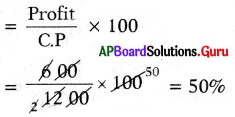∴ Profit percentage = 50%

Question 7.
After incurring a loss of ₹ 258, Jean pant was sold at ₹ 1750. Then find the cost price and loss percentage?
Given selling price of Jean pant = ₹ 1750
Loss = ₹ 258
Cost price of Jean pant = S.P + Loss
= 1750 + 258
= ₹ 2008
Loss percent =  Loss  C.P  × 100
⇒ 2582008 × 100
∴ Loss percentage = 12.85%

Question 8.
The cost price of 10 articles is equal to the selling price of 9 articles. Find the profit percentage?
Method 1:
Let Cost price of 10 articles be ₹ x.
Cost price of 10 articles = 10 x
Selling price of 9 articles = 10 x
Cost price of 9 articles 9x
Profit = S.P — C.P = 10x – 9x
= ₹ x
Profit percentage =  Profit  C.P  × 100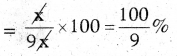∴ Profit percentage = 1009% (or)
11.11% (or) 1119%

Method 2:
By selling 9 articles, there is a gain of 1 article.
∴ Profit Percentage = 19 × 100
= 1119%

Question 9.
By selling a book for ₹ 258, a bookseller gains 20%. For how much should he sell it to gain 30%?
Method 1:
Given selling priceof a book = ₹ 258
Gain = 20%
Cost price of book = ₹ x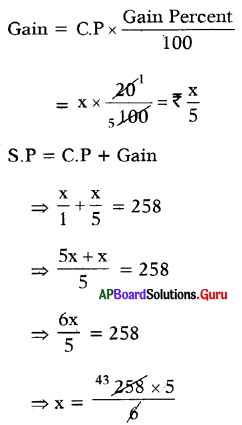∴ Cost price of a book = ₹ 215

If profit percent = 30%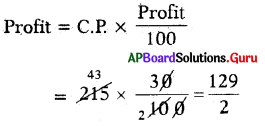Profit = ₹ 64.50
∴ S.P = C.P + Profit
= 215 + 64.50 = 279.50
To get 30% profit he has to sold at ₹ 279.50.

Method 2:
Let the C.P of the book = 100%
Selling at a gain of 20% means (100 + 20)% = ₹ 258
120% = ₹ 258
Selling at a gain of 30% means (100+30)%=?
If 120% = ₹ 258
then 130% =?
∴ SP = 130120 × 258 = ₹ 279.5

## Andhra Pradesh Board Class 7th Maths Chapter 7 Ratio and Proportion Ex 7.5 Textbooks for Exam Preparations

Andhra Pradesh Board Class 7th Maths Chapter 7 Ratio and Proportion Ex 7.5 Textbook Solutions can be of great help in your Andhra Pradesh Board Class 7th Maths Chapter 7 Ratio and Proportion Ex 7.5 exam preparation. The AP Board STD 7th Maths Chapter 7 Ratio and Proportion Ex 7.5 Textbooks study material, used with the English medium textbooks, can help you complete the entire Class 7th Maths Chapter 7 Ratio and Proportion Ex 7.5 Books State Board syllabus with maximum efficiency.

## FAQs Regarding Andhra Pradesh Board Class 7th Maths Chapter 7 Ratio and Proportion Ex 7.5 Textbook Solutions

#### Can we get a Andhra Pradesh State Board Book PDF for all Classes?

Yes you can get Andhra Pradesh Board Text Book PDF for all classes using the links provided in the above article.

## Important Terms

Andhra Pradesh Board Class 7th Maths Chapter 7 Ratio and Proportion Ex 7.5, AP Board Class 7th Maths Chapter 7 Ratio and Proportion Ex 7.5 Textbooks, Andhra Pradesh State Board Class 7th Maths Chapter 7 Ratio and Proportion Ex 7.5, Andhra Pradesh State Board Class 7th Maths Chapter 7 Ratio and Proportion Ex 7.5 Textbook solutions, AP Board Class 7th Maths Chapter 7 Ratio and Proportion Ex 7.5 Textbooks Solutions, Andhra Pradesh Board STD 7th Maths Chapter 7 Ratio and Proportion Ex 7.5, AP Board STD 7th Maths Chapter 7 Ratio and Proportion Ex 7.5 Textbooks, Andhra Pradesh State Board STD 7th Maths Chapter 7 Ratio and Proportion Ex 7.5, Andhra Pradesh State Board STD 7th Maths Chapter 7 Ratio and Proportion Ex 7.5 Textbook solutions, AP Board STD 7th Maths Chapter 7 Ratio and Proportion Ex 7.5 Textbooks Solutions,
Share: